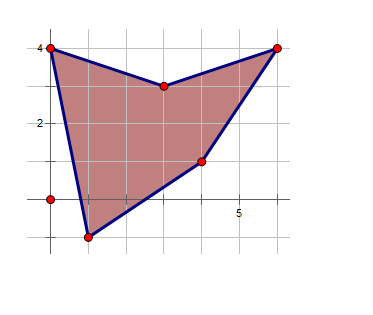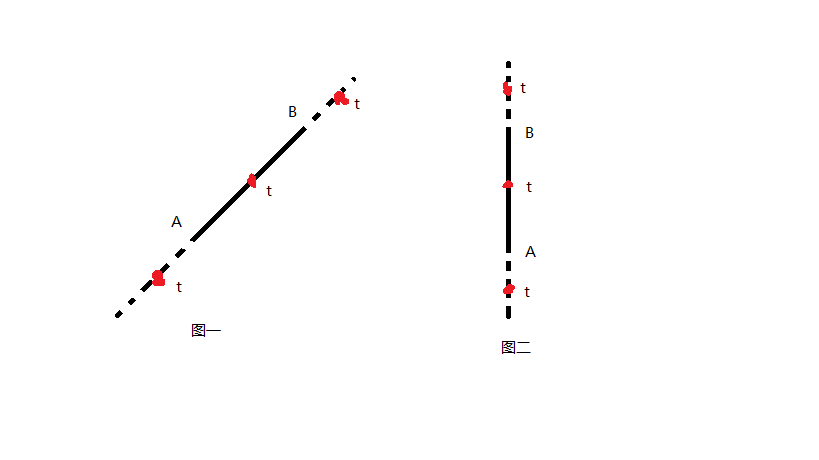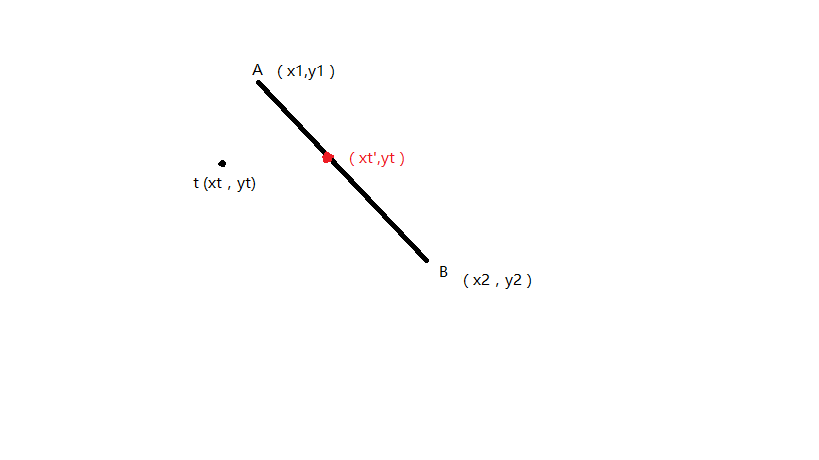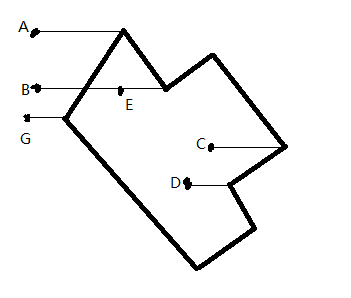# 广场舞 （第七届蓝桥杯决赛题过50%数据讲解代码+过100%数据思路）

LQ市的市民广场是一个多边形，广场上铺满了大理石的地板砖。

（广场形状的例子参考【图1.png】）【输入格式】

【输出格式】

【样例输入】
5
3 3
6 4
4 1
1 -1
0 4

【样例输出】
7

【样例说明】

【数据规模与约定】

CPU消耗  < 1000ms1：图一所示枚举的这条边有斜率的情况下，我们先求出这条边的斜率，在求出测试点和一个顶点的斜率，如果斜率相同就有图一三种情况，在线段上一种和在线段延长线上两种，只有在线段上才是在多边形内，所以斜率相同并且t的x横坐标在两个端点x横坐标之间的时候是在形内的。

2：图二所示枚举的边斜率无穷大，就是三个点x值相同时，说明斜率无穷大，然后只需让t的y值在两个端点的y值之间就可保证在形内(vy[i] > testy) != (vy[j] > testy)，也就是一个端点的y大于测试点的y（值为1）一个端点的y小于等于测试点的y（值为0）这样就满足的！=，这样就是真1，判断就完成了，不用麻烦的分类了。

#include <iostream>
#include <cstdio>
#include <cstring>
using namespace std;
//待输入点的坐标
double vx,vy;
int num;//顶点个数

//关键函数，判断点是否在凸多边形内
int isIn(double testx,double testy){
for(int k = 0; k < num; k++){
if(testx == vx[k] && testy == vy[k])//这个点恰好是顶点，当然算在里面
return 1;
}

for(int i = 0; i < num; i++){
double dy = vy[(i+1)%num] - vy[i];
double dx = vx[(i+1)%num] - vx[i];
if(dx == 0.0) continue;
double k = dy / dx;//算出多边形某条面的斜率
if((vy[i] - testy) / (vx[i]-testx) == k && (testx > vx[i]) != (testx > vx[(i+1)%num]))
return 1;
//上面的判断什么意思呢？我们来分析一下，首先发现这个测试点和边上一点的斜率和这条边斜率相同说明什么
//这保证了这个点必定在这条线段上或者线段的延长线上，那么只有当在线段上才是在图形内部
//所以只需要让测试点的x横坐标在线段连端点的x横坐标之间即可，上面写法的意思就是不能在同侧
//测试点x值不能同时在两端点左侧，也不能同时在两端右侧，而在某一个点上的情况之前就已经判断了
}

//上面for循环判断了在边上的情况，我们用到了斜率，但是有一种斜率是求不出来的,就是和y轴平行的时候下面就是对这种情况的判断
for(int i = 0; i < num; i++){
if(testx == vx[i] && vx[i] == vx[(i+1)%num] && (testy > vy[i]) != (testy > vy[(i+1)%num]))
return 1;
//三点x横坐标相等，测试点的y值在两端点之间
}

//以上点在边上的情况就判断完了，下面不在边上的情况我们就利用水平射线相交点判别法判断
int flag = 0;//记录交点个数的奇偶性
for(int i = 0,j = num-1; i < num; j = i++){
if((vy[i] > testy) != (vy[j] > testy) && (testx < (vx[j] - vx[i]) * (testy - vy[i]) / (vy[j] - vy[i]) + vx[i]))
flag = !flag;
}
return flag;
//具体解释见上面博客的解释
}

bool IsSquare(double x,double y){
//判断是否可以构成小正方形及判断它本身和它的左，下，左下点是否在凸多边形内
if(isIn(x,y)){
if(!isIn(x+1.0,y)) return false;
if(!isIn(x,y-1.0)) return false;
if(!isIn(x+1.0,y-1.0)) return false;
return true;
}
else
return false;
}

int main(){
int ans = 0;
scanf("%d",&num);
for(int i = 0; i < num; i++){
scanf("%lf%lf",&vx[i],&vy[i]);
}
//获得图形的边界
double xmax = vx,xmin = vx;
double ymax = vy,ymin = vy;
for(int i = 0; i < num; i++){
xmax = max(xmax,vx[i]);
xmin = min(xmin,vx[i]);
ymax = max(ymax,vy[i]);
ymin = min(ymin,vy[i]);

}

//扫描范围内的每个点
for(double x = xmin; x <= xmax; x++){
for(double y = ymax; y >= ymin; y--){
//判断是否可以构成一个完整的小正方
if(IsSquare(x,y)) ans++;
}
}
printf("%d\n",ans);
return 0;
}